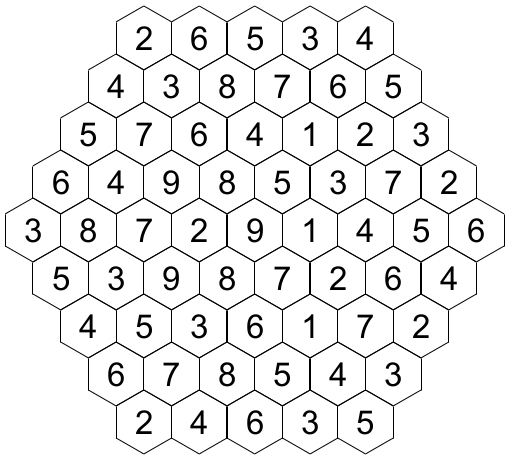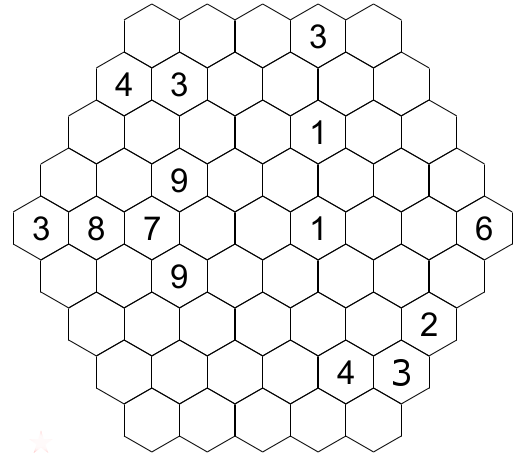Hanidoku

## Rules of Haniddoku

Hanidoku is a different puzzle, first the grid and the cells  have a shape of a hexagon.  Each cell is at the intersection of one row and two diagonals (ascending and descending). The rows and diagonals have different sizes (from 5 to 9). The goal is to put number 1 to 9 into the cells so that each row and diagonal contains different numbers but also the numbers in each row and diagonal must form a sequence of consecutive numbers. So this is a gridand the solution.## Goal

To put numbers ( 1to 9) in each cell such that at the end each row and diagonal has different numbers and that the number form a sequence of consecutive numbers. The sequence mut never has a hole ex. in a row of size 5  the sequence 3,4,5,7,8 is not valid because it has a hole between the 5 and 7 (no 6).

In a row or diagonal of size 5 the only valid sequences are : 12345, 23456,34567,45678 et 56789. The common number of each possible sequence is 5, so all the other numbers are optional. The following table shows for all size the possible sequences.

 Size Possible sequences Common Optional 5 12345,23456,34567,45678,56789 5 1,2,3,4,6,7,8,9 6 123456,234567,345678,456789 4,5,6 1,2,3,7,8,9 7 1234567,2345678,3456789 3,4,5,6,7 1,2,8,9 8 12345678,23456789 2,3,4,5,6,7,8 1,9 9 123456789 1,2,3,4,5,6,7,8,9

This means that for a row of size 7, it must have 3,4,5,6,7 and the two other numbers could be 1,2,8 or 9, but don't forget it must form a sequence of consecutive numbers so if the 1 appears then the 2 must be present else the sequence is not consecutive. The number 5 is present in each row and diagonal, the numbers 4 and 6 are necessary present in row or digaional of size 6 and more, but they could appear also in row or diagonal of size 5.I present you some strategies to resolve Hanidoku.

## Technique for resolving Hanidoku

### Elimination

We must eliminate in a cell the numbers present in the other cells of the row and the two diagonals. Like as Sudoku you must eliminate numbers in cell that are present in the other cells of the row,column and region.

### Gap

One of the challenge is to determine wich number are present in a row or diagonal of size 8 and less. So if a row or diagional contains at least two number so this row must contain at least all the numbers between the two numbers ex in a row of size 5, a cell has a 4 and another cell has a 8 so the number 5,6 and 7 must be in the other cells of the row. If a row or diagonal contains a 1 or a 9 you don't need the second number to determine the possible numbers.

Application of these two techniquesIn the ascending diagona of size 5  (at the right) it has a 6 and a 2. So by the method of the gap the missing numbers are  3,4 et 5.We can eliminat the num,ber 3 in the green cells because the diagonals touching this diagonal (in green)  have already a 3. So the number 3 must go in the white cell (next figure).  As an exercice you could try to put the 4 in this diagonal the answer is the full grid at the  top of the page.### Triangulation

You will need this technique to resolve puzzles with 4 stars and more, but nothing prevents you to use it in easier puzzles. If in a row or diagonal only two cells ( and only twos)  could have a candidate (and no other cells of the row could accept this candidate) then we can eliminate this candidate in the cell at the intersection, by the diagonals, of these two cells.For illustrating this method I give you an exemple. Suppose that in last diagonal, it have only  two cells (in yellow) in this diagonal  that could contain the number 2 and 5 (naked pair), It iis important to specify that the 2 and 5 cannot go elsewhere in the diagonal. In this case, because it is a naked pai,r we can eliminate the 2 and 5 from the other cells of the diagonal ( but most of the time it will be only one number (candidate) that could be placed in 2 cells). So we could eliminate the candidate 5 in the green cell  at the intersection by the diagonal of the two yellow cells  So the green cell could only accept the 4.

I believe that you have enough knowledge to have fun with Hanidoku.